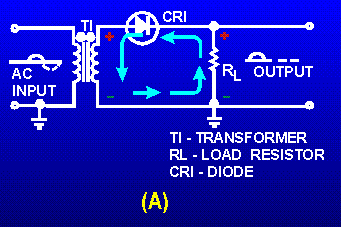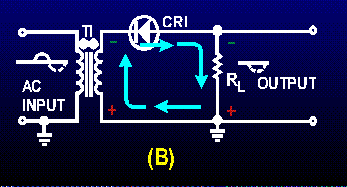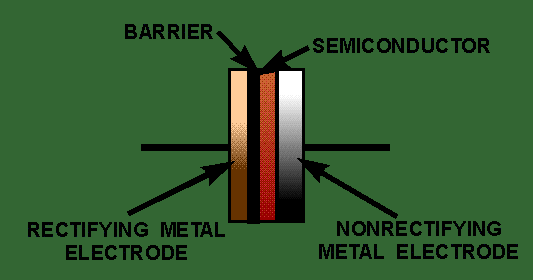doping agents, semiconductor materials, and manufacturing techniques have made it possible to produce diodes that can be used in many different applications. Examples of these types of diodes are signal diodes, rectifying diodes, Zener diodes (voltage protection diodes for power supplies), varactors (amplifying and switching diodes), and many more.">Custom SearchUntil now, we have mentioned only one application for the diode-rectification, but there are many more applications that we have not yet discussed. Variations in doping agents, semiconductor materials, and manufacturing techniques have made it possible to produce diodes that can be used in many different applications. Examples of these types of diodes are signal diodes, rectifying diodes, Zener diodes (voltage protection diodes for power supplies), varactors (amplifying and switching diodes), and many more. Only applications for two of the most commonly used diodes, the signal diode and rectifier diode, will be presented in this chapter. The other diodes will be explained later on in this module. Half-Wave Rectifier One of the most important uses of a diode is rectification. The normal PN junction diode is well-suited for this purpose as it conducts very heavily when forward biased (low-resistance direction) and only slightly when reverse biased (high-resistance direction). If we place this diode in series with a source of ac power, the diode will be forward and reverse biased every cycle. Since in this situation current flows more easily in one direction than the other, rectification is accomplished. The simplest rectifier circuit is a half-wave rectifier (fig. 1-21 view A and view B) which consists of a diode, an ac power source, and a load resister. Figure 1-21A. - Simple half-wave rectifier.Figure 1-21B. - Simple half-wave rectifier.The transformer (T1) in the figure provides the ac input to the circuit; the diode (CR1) provides the rectification; and the load resistor (RL) serves two purposes: it limits the amount of current flow in the circuit to a safe level, and it also develops the output signal because of the current flow through it. Before describing how this circuit operates, the definition of the word "load" as it applies to power supplies must be understood. Load is defined as any device that draws current. A device that draws little current is considered a light load, whereas a device that draws a large amount of current is a heavy load. Remember that when we speak of "load," we are speaking about the device that draws current from the power source. This device may be a simple resistor, or one or more complicated electronic circuits. During the positive half-cycle of the input signal (solid line) in figure 1-21 view A, the top of the transformer is positive with respect to ground. The dots on the transformer indicate points of the same polarity. With this condition the diode is forward biased, the depletion region is narrow, the resistance of the diode is low, and current flows through the circuit in the direction of the solid lines. When this current flows through the load resistor, it develops a negative to positive voltage drop across it, which appears as a positive voltage at the output terminal. When the ac input goes in a negative direction (fig. 1-21 view A), the top of the transformer becomes negative and the diode becomes reverse biased. With reverse bias applied to the diode, the depletion region increases, the resistance of the diode is high, and minimum current flows through the diode. For all practical purposes, there is no output developed across the load resistor during the negative alternation of the input signal as indicated by the broken lines in the figure. Although only one cycle of input is shown, it should be realized that the action described above continually repeats itself, as long as there is an input. Therefore, since only the positive half-cycles appear at the output this circuit converted the ac input into a positive pulsating dc voltage. The frequency of the output voltage is equal to the frequency of the applied ac signal since there is one pulse out for each cycle of the ac input. For example, if the input frequency is 60 hertz (60 cycles per second), the output frequency is 60 pulses per second (pps). However, if the diode is reversed as shown in view B of figure 1-21, a negative output voltage would be obtained. This is because the current would be flowing from the top of RL toward the bottom, making the output at the top of RL negative with respect to the bottom or ground. Because current flows in this circuit only during half of the input cycle, it is called a half-wave rectifier. The semiconductor diode shown in the figure can be replaced by a metallic rectifier and still achieve the same results. The metallic rectifier, sometimes referred to as a dry-disc rectifier, is a metal-to-semiconductor, large-area contact device. Its construction is distinctive; a semiconductor is sandwiched between two metal plates, or electrodes, as shown in figure 1-22. Note in the figure that a barrier, with a resistance many times greater than that of the semiconductor material, is constructed on one of the metal electrodes. The contact having the barrier is a rectifying contact; the other contact is nonrectifying. Metallic rectifiers act just like the diodes previously discussed in that they permit current to flow more readily in one direction than the other. However, the metallic rectifier is fairly large compared to the crystal diode as can be seen in figure 1-23. The reason for this is: metallic rectifier units are stacked (to prevent inverse voltage breakdown), have large area plates (to handle high currents), and usually have cooling fins (to prevent overheating). Figure 1-22. - A metallic rectifier.Figure 1-23. - Different types of crystal and metallic rectifiers.There are many known metal-semiconductor combinations that can be used for contact rectification. Copper oxide and selenium devices are by far the most popular. Copper oxide and selenium are frequently used over other types of metallic rectifiers because they have a large forward current per unit contact area, low forward voltage drop, good stability, and a lower aging rate. In practical application, the selenium rectifier is used where a relatively large amount of power is required. On the other hand, copper-oxide rectifiers are generally used in small-current applications such as ac meter movements or for delivering direct current to circuits requiring not more than 10 amperes. Since metallic rectifiers are affected by temperature, atmospheric conditions, and aging (in the case of copper oxide and selenium), they are being replaced by the improved silicon crystal rectifier. The silicon rectifier replaces the bulky selenium rectifier as to current and voltage rating, and can operate at higher ambient (surrounding) temperatures. Diode Switch In addition to their use as simple rectifiers, diodes are also used in circuits that mix signals together (mixers), detect the presence of a signal (detector), and act as a switch "to open or close a circuit." Diodes used in these applications are commonly referred to as "signal diodes." The simplest application of a signal diode is the basic diode switch shown in figure 1-24. Figure 1-24. - Basic diode switch.When the input to this circuit is at zero potential, the diode is forward biased because of the zero potential on the cathode and the positive voltage on the anode. In this condition, the diode conducts and acts as a straight piece of wire because of its very low forward resistance. In effect, the input is directly coupled to the output resulting in zero volts across the output terminals. Therefore, the diode, acts as a closed switch when its anode is positive with respect to its cathode. If we apply a positive input voltage (equal to or greater than the positive voltage supplied to the anode) to the diode's cathode, the diode will be reverse biased. In this situation, the diode is cut off and acts as an open switch between the input and output terminals. Consequently, with no current flow in the circuit, the positive voltage on the diode's anode will be felt at the output terminal. Therefore, the diode acts as an open switch when it is reverse biased. Q.27 What is a load?Q.28 What is the output of a half-wave rectifier?Q.29 What type of rectifier is constructed by sandwiching a section of semiconductor material between two metal plates?Q.30 What type of bias makes a diode act as a closed switch?Integrated Publishing, Inc. - A (SDVOSB) Service Disabled Veteran Owned Small Business